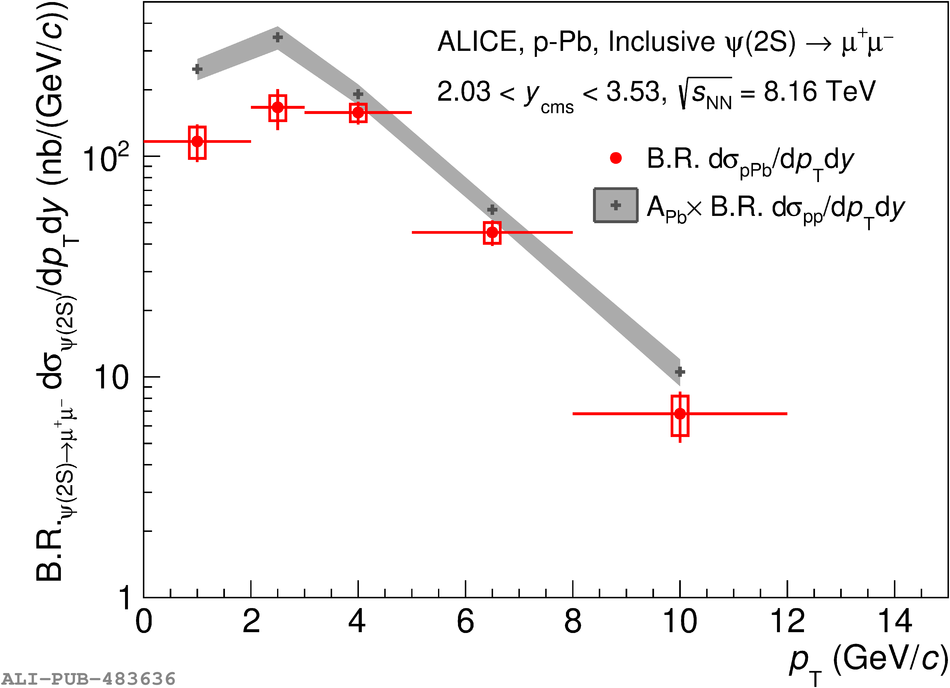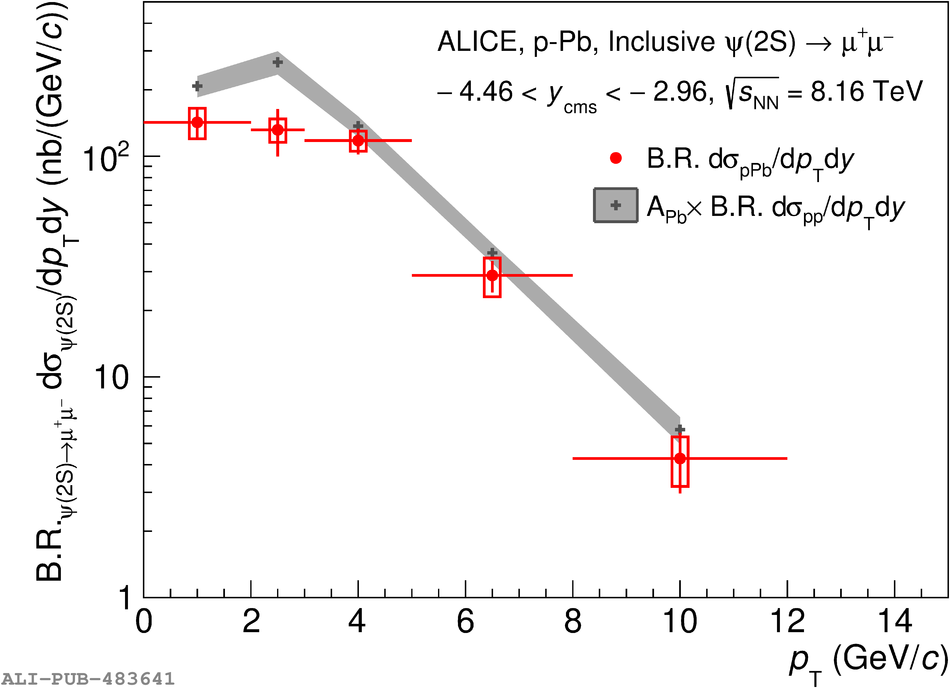# Figure 3

 The differential cross sections ${\rm B.R.}_{\psi(2{\rm S})\rightarrow\mu^+\mu^-}{\rm d}^2\sigma^{\psi(2{\rm S})}/{\rm d}y{\rm d}p_{\rm T}$ for \ppb collisions at $\sqrtSnn = 8.16$ TeV, shown separately for the forward and backward $y_{\rm cms}$ samples. The error bars represent the statistical uncertainties, while the boxes correspond to total systematic uncertainties. The latter are uncorrelated among the points, except for a very small correlated uncertainty (0.5\% and 0.7\% for the forward and backward $y_{\rm cms}$ samples, respectively). The grey bands correspond to the reference pp cross section scaled by A$_{\rm Pb}$.